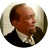Can I use the sum of values in columns a chart categories?

Can I use the sum or other aggreagations of the values in the columns as categories in pie and bar charts?

For example, a pie chart will have 2 categories whose value will be the sum of two columns.

I can't figure out what the data displayed in the charts actually is. How is the data aggregated and how do I chage it? The documentation does not really explain very clearly. Right how, for a pie chart, I select the two columns, choose SUM as the Footer (?) OR use the sum function. What I get is a pie wedge for each value in my column. It does not even aggregate. If a column has the number 15 in it eight times, I get 8 wedges labeled 15. I would at least expect each wedge to represent the number of instances of each category.This is possible. You can group by column and display the the Sum in a number column.

Birger